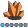fred777
Calcite | Level 5

## IF (in data step) versus WHERE (in PROC)

blah is a character variable mostly populated with numbers in dataset fred.

if I do this ...

data fred2;

set fred;

if sum(blah) ^= .;

run;

... SAS will give me just those records which don't have just numbers in blah.

However, if I try something like this ...

proc tabulate data=fred;

where sum(blah) ^=.;
.

.

.

run;

... SAS complains with the error:
ERROR: Function SUM requires a numeric expression as argument 1.

Is there any way I can avoid that additional data step above and still filter on sum(blah) ^=. directly in the PROC?

## Re: IF (in data step) versus WHERE (in PROC)

The DATA step code is actually compiled, and not just run from a precompiled module as in PROC steps.

Therefore the SAS datastep compiler can recognize variable type inconsistencies and compile a typecast into the code. This is what causes the NOTE: messages about type conversion in the log.

This is, of course, not possible for dataset options (the where condition in the PROC is working like a dataset option) that don't have the luxury of compilation.

So you won't get around the character-to-numeric conversion, be it explicit or implicit.

Discussion stats